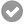# Base Report. Time difference function

My DB has two time fields StartTime, EndTime, both of which occur on the same day.

If I make a function in the ORB

[EndTime]

a text field with that function prints the end time exactly as expected.

A function with [EndTime]-[StartTime] prints all zeros.

Making a Query with [EndTime]-[StartTime] works as expected, printing the difference in hours.

What is the proper formatting for this function?

I suggest extracting the hour and minute and doing the calculation without a built-in function. In short, you can get the minute difference with this:

`````` ( HOUR( "EndTime" ) - HOUR( "StartTime" ) ) * 60 + ( MINUTE( "EndTime" ) - MINUTE( "StartTime" ) )
``````

For a formatted ‘hh:mm’ output, this query does a little more work:

``````SELECT "StartTime", "EndTime",
CONCAT( CONCAT( "hours", ':' ), ( "minutes" - ( "hours" * 60 ) ) ) "DiffTime"
FROM (
SELECT "PriKey", "StartTime", "EndTime", ( HOUR( "EndTime" ) - HOUR( "StartTime" ) ) * 60 +
( MINUTE( "EndTime" ) - MINUTE( "StartTime" ) ) "minutes",
( ( HOUR( "EndTime" ) - HOUR( "StartTime" ) ) * 60
+ ( MINUTE( "EndTime" ) - MINUTE( "StartTime" ) ) ) / 60 "hours"
FROM "Table1"
) AS "subquery"
``````

Alternatively, if your variables are `DATETIME` and not just `TIME`, you can use the `DATEDIFF` function documented in the HSQLDB v1.8 manual.

There are other ways to accomplish this result also, for example, in a `Basic` macro.

EDIT: Renamed the fields in the second query to be consistent with the first.

(if this answered your question, please accept answer by clicking check mark () to the left)

[comment deleted]

This SQL incorrectly configures the minutes when end is less than start.
See Base Query SQL Time difference problems - #11 by FKlusmann
I have learned that Base, HSQLDB, and Firebird handle DATE, TIME, and INTERVAL as the same. Therefore one needs to look for DATE operations to work with TIME. @Ratslayer provided solutions for Firebird in the link above. This works for HSQLDB and shows my thoughts.
` /* Q_Time_Differences (on the same day) */
SELECT “PriKey”, “StartTime”, “EndTime”,

DATEDIFF(‘MINUTE’,“StartTime”,“EndTime”) / 60 AS “LapsedHrs”,
DATEDIFF(‘MINUTE’,“StartTime”,“EndTime”) AS “LapsedMins”,
DATEDIFF(‘MINUTE’,“StartTime”,“EndTime”) / 60.00 AS “LapsedHrsDecimal”,

(continued below)

(from above)

/* LapsedMins - LapsedHrs * 60 /
(DATEDIFF(‘MINUTE’,“StartTime”,“EndTime”)) - ((DATEDIFF(‘MINUTE’,“StartTime”,“EndTime”) / 60) * 60) AS “MoreMins”,
/
LapsedHrs pipes : pipes MoreMins */
(DATEDIFF(‘MINUTE’,“StartTime”,“EndTime”) / 60) || ‘:’ || ((DATEDIFF(‘MINUTE’,“StartTime”,“EndTime”)) - ((DATEDIFF(‘MINUTE’,“StartTime”,“EndTime”) / 60) * 60)) As “ElapsedTime”
FROM “Table1”